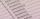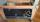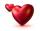Pets in ZOO

The zoo have run for pets. They are chickens, pigs and cow. Altogether there has 20 heads and 60 feet. How many chickens and piglets are there?

Result

x =  10
y =  9

Solution:

x+y+1 = 20
2x+4y+4=60

x+y = 19
2x+4y = 56

x = 10
y = 9

Calculated by our linear equations calculator.

Leave us a comment of this math problem and its solution (i.e. if it is still somewhat unclear...):Be the first to comment!To solve this verbal math problem are needed these knowledge from mathematics:

Do you have a linear equation or system of equations and looking for its solution? Or do you have quadratic equation?

Next similar math problems:

1. School marksBoris has a total of 22 marks. Ones have 3 times less than threes. There are two twos. How many has ones and threes?
2. Jan and DanJan and Dan had the same money. Jan bought 5 workbooks and left him 15 CZK. Dan 6 and left him nothing. How much money have in total?
3. Forest nurseryIn the forest nursery after winter, they found that 1/10 stems died out of them. For them, they land 193 new spruces. How many spruces are in the forest nursery?
4. Foot in busIt was 102 people on the bus. 28 girls had two dogs. A 11 girls had one dog. At the next stop seceded 5 dogs (even with their owners). They got two boys together with three dogs. The bus drove one driver. How many foot were in bus?
5. HotelThe rooms in the mountain hotel are double and triple. Double rooms are 25 and triple are 17 more. How many rooms are there in this hotel?
6. CagesHonza had three cages (black, silver, gold) and three animals (guinea pig, rat and puppy). There was one animal in each cage. The golden cage stood to the left of the black cage. The silver cage stood on the right of the guinea pig cage. The rat was in the
7. PopsiclesFrancis went to buy ice lollies. If he buy 8 popsicles he missed 4 USD. When he buy 7 popsicles, got back 1 USD. How many USD was a popsicle?
8. SnacksThe school attends 344 pupils. Half of them take snacks. 13 pupils who took snacks did not attend school. How many snacks left?
9. Apples 3Julka has 5 apples more than Hugo and four apples less than Annie. Hugo has 17 apples. How many apples has Julka and how Annie?
10. Mom and dadMom wants talk with dad 2.2 hours. But dad wants of 2 times more hours than mom ... How many hours wants dad talk with mom? ...
11. BenchesThe park has 64 benches. Occupied are by 18 more than empty. How many benches are occupied and empty ?
12. Unknown number xyzFind the number that its triple is 24. Solve by equation.
13. Eq1Solve equation: 4(a-3)=3(2a-5)
14. Six te 2If 3t-7=5t, then 6t=
15. Addition of Roman numbersAdded together and write as decimal number: LXVII + MLXIV
16. Simple equation 8Solve the following equation: 36=-(1+7x)-6(-7-x)
17. If-then equationIf 5x - 17 = -x + 7, then x =